Test: Statistical Description Of Data - 2

# Test: Statistical Description Of Data - 2

Test Description

## 40 Questions MCQ Test Business Mathematics and Logical Reasoning & Statistics | Test: Statistical Description Of Data - 2

Test: Statistical Description Of Data - 2 for CA Foundation 2023 is part of Business Mathematics and Logical Reasoning & Statistics preparation. The Test: Statistical Description Of Data - 2 questions and answers have been prepared according to the CA Foundation exam syllabus.The Test: Statistical Description Of Data - 2 MCQs are made for CA Foundation 2023 Exam. Find important definitions, questions, notes, meanings, examples, exercises, MCQs and online tests for Test: Statistical Description Of Data - 2 below.
Solutions of Test: Statistical Description Of Data - 2 questions in English are available as part of our Business Mathematics and Logical Reasoning & Statistics for CA Foundation & Test: Statistical Description Of Data - 2 solutions in Hindi for Business Mathematics and Logical Reasoning & Statistics course. Download more important topics, notes, lectures and mock test series for CA Foundation Exam by signing up for free. Attempt Test: Statistical Description Of Data - 2 | 40 questions in 40 minutes | Mock test for CA Foundation preparation | Free important questions MCQ to study Business Mathematics and Logical Reasoning & Statistics for CA Foundation Exam | Download free PDF with solutions
 1 Crore+ students have signed up on EduRev. Have you?
Test: Statistical Description Of Data - 2 - Question 1

### (Direction 1 - 20) Answer the following questions. Each question carries 1 mark. Q. Pie-diagram is used for

Test: Statistical Description Of Data - 2 - Question 2

### A frequency distribution

Test: Statistical Description Of Data - 2 - Question 3

### The frequency distribution of a continuous variable is known as

Test: Statistical Description Of Data - 2 - Question 4

The distribution of shares is an example of the frequency distribution of

Test: Statistical Description Of Data - 2 - Question 5

The distribution of profits of a blue-chip company relates to

Test: Statistical Description Of Data - 2 - Question 6

Analysis based on study of price fluctuations, production of commodities and deposits in banks is classified as

Test: Statistical Description Of Data - 2 - Question 7

Mutually inclusive classification is usually meant for

Test: Statistical Description Of Data - 2 - Question 8

Mutually exclusive classification is usually meant for

Test: Statistical Description Of Data - 2 - Question 9

The LCB is

Test: Statistical Description Of Data - 2 - Question 10

Branch of statistics which deals with development of particular statistical methods is classified as

Test: Statistical Description Of Data - 2 - Question 11

length of a class is

Test: Statistical Description Of Data - 2 - Question 12

For a particular class boundary, the less than cumulative frequency and more than cumulative frequency add up to

Test: Statistical Description Of Data - 2 - Question 13

Frequency density corresponding to a class interval is the ratio of

Test: Statistical Description Of Data - 2 - Question 14

Relative frequency for a particular class

Test: Statistical Description Of Data - 2 - Question 15

​Mode of a distribution can be obtained from

Test: Statistical Description Of Data - 2 - Question 16

Median of a distribution can be obtained from

Test: Statistical Description Of Data - 2 - Question 17

A comparison among the class frequencies is possible only in

Test: Statistical Description Of Data - 2 - Question 18

Frequency curve is a limiting form of

Test: Statistical Description Of Data - 2 - Question 19

Most of the commonly used frequency curves are

Test: Statistical Description Of Data - 2 - Question 20

The distribution of profits of a company follows

Test: Statistical Description Of Data - 2 - Question 21

(Direction 21 - 27) Answer the following questions. Each question carries 2 marks.

Q. Out of 1000 persons, 25 per cent were industrial workers and the rest were agricultural workers. 300 persons enjoyed world cup matches on TV. 30 per cent of the people who had not watched world cup matches were industrial workers. What is the number of agricultural workers who had enjoyed world cup matches on TV?

Test: Statistical Description Of Data - 2 - Question 22

A sample study of the people of an area revealed that total number of women were 40% and the percentage of coffee drinkers were 45 as a whole and the percentage of male coffee drinkers was 20. What was the percentage of female non-coffee drinkers?

Test: Statistical Description Of Data - 2 - Question 23

Cost of sugar in a month under the heads Raw Materials, labour, direct production and others were 12, 20, 35 and 23 units respectively. What is the difference between the central angles for the largest and smallest components of the cost of sugar?

Test: Statistical Description Of Data - 2 - Question 24

The number of accidents for seven days in a locality are given below :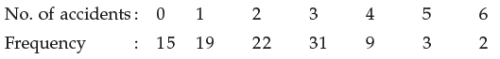Q. What is the number of cases when 3 or less accidents occurred?

Test: Statistical Description Of Data - 2 - Question 25

The following data relate to the incomes of 86 persons :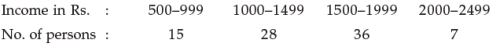Q. What is the percentage of persons earning more than Rs. 1500?

Test: Statistical Description Of Data - 2 - Question 26

The following data relate to the marks of a group of students: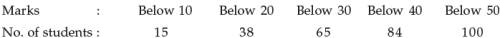Q. How many students got marks more than 30?

Test: Statistical Description Of Data - 2 - Question 27

In which files, the records are organised in sequence and an index table is used to speed up access to the records without requiring a search of the entire file?

Test: Statistical Description Of Data - 2 - Question 28

(Direction 28 - 32) Answer the following questions. Each question carries 5 marks.

In a study about the male and female students of commerce and science departments of a college in 5 years, the following datas were obtained :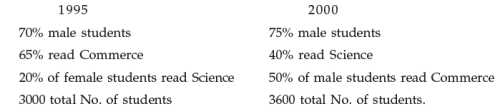Q. After combining 1995 and 2000 if x denotes the ratio of female commerce student to female Science student and y denotes the ratio of male commerce student to male Science student, then

Detailed Solution for Test: Statistical Description Of Data - 2 - Question 28

The correct option is Option A.

1995

70% male students = 2100 (70% of 3000)

30% female students = 900

20% of female read science = 180

80% of female read commerce = 720

Male students read commerce = 1230 (1950 - 720)

Male students read science = 870

2000

75% male students = 2700 (75% of 3600)

25% female students = 900

50% of male students read commerce = 1350

50% of male students read science = 1350

Female students of science = 90

Female students of commerce = 810

x = female commerce students / female science students

= 720 + 810 / 180 + 90

= 1530 / 270

= 5.666

y = male commerce students / female commerce students

= 1230 + 1350 / 870 + 1350

= 2580 / 2220

= 1.162

So, x > y

Hence proved

Test: Statistical Description Of Data - 2 - Question 29

In a study relating to the labourers of a jute mill in West Bengal, the following information was collected.

‘Twenty per cent of the total employees were females and forty per cent of them were married. Thirty female workers were not members of Trade Union. Compared to this, out of 600 male workers 500 were members of Trade Union and fifty per cent of the male workers were married. The unmarried non-member male employees were 60 which formed ten per cent of the total male employees. The unmarried non-members of the employees were 80’. On the basis of this information, the ratio of married male non-members to the married female non-members is

Test: Statistical Description Of Data - 2 - Question 30

The weight of 50 students in pounds are given below :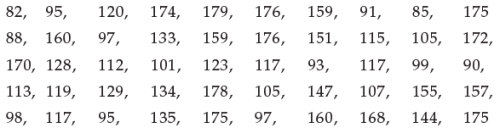Q. If the data are arranged in the form of a frequency distribution with class intervals as 81-100, 101-120, 121-140, 141-160 and 161-180, then the frequencies for these 5 class intervals are

Test: Statistical Description Of Data - 2 - Question 31

The following data relate to the marks of 48 students in statistics :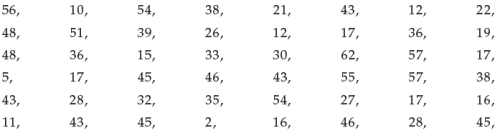Q. What are the frequency densities for the class intervals 30-39, 40-49 and 50-59

Test: Statistical Description Of Data - 2 - Question 32

The following information relates to the age of death of 50 persons in an area :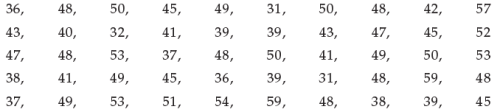Q. If the class intervals are 31-33, 34-36, 37-39, …. Then the percentage frequencies for the last five class intervals are

Detailed Solution for Test: Statistical Description Of Data - 2 - Question 32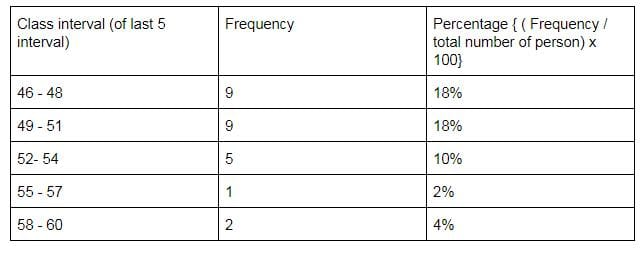Test: Statistical Description Of Data - 2 - Question 33

Graph is a

Test: Statistical Description Of Data - 2 - Question 34

​Details are shown by

Test: Statistical Description Of Data - 2 - Question 35

The relationship between two variables are shown in

Test: Statistical Description Of Data - 2 - Question 36

In general the number of types of tabulation are

Test: Statistical Description Of Data - 2 - Question 37

Statistics branches include

Detailed Solution for Test: Statistical Description Of Data - 2 - Question 37

The two main branches of statistics are descriptive statistics and inferential statistics. Both of these are employed in scientific analysis of data and both are equally important for the student of statistics.

Test: Statistical Description Of Data - 2 - Question 38

The number of errors in Statistics are

Test: Statistical Description Of Data - 2 - Question 39

The number of “Frequency distribution“ is

Test: Statistical Description Of Data - 2 - Question 40

(Class frequency)/(Width of the class ) is defined as

## Business Mathematics and Logical Reasoning & Statistics

131 videos|149 docs|87 tests
 Use Code STAYHOME200 and get INR 200 additional OFF Use Coupon Code
Information about Test: Statistical Description Of Data - 2 Page
In this test you can find the Exam questions for Test: Statistical Description Of Data - 2 solved & explained in the simplest way possible. Besides giving Questions and answers for Test: Statistical Description Of Data - 2, EduRev gives you an ample number of Online tests for practice

## Business Mathematics and Logical Reasoning & Statistics

131 videos|149 docs|87 tests